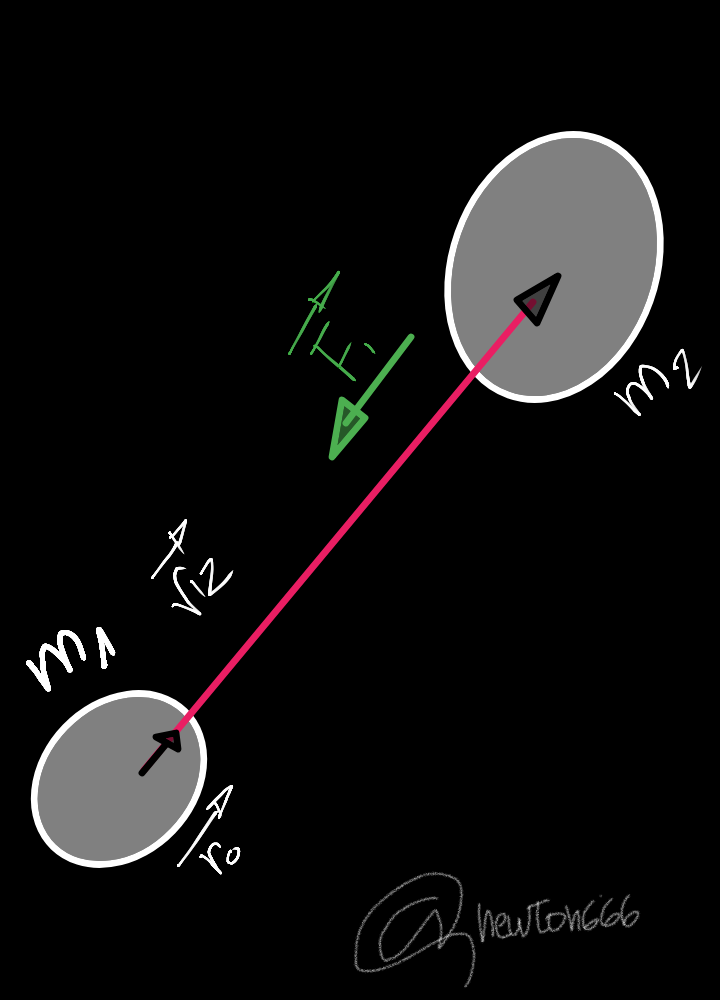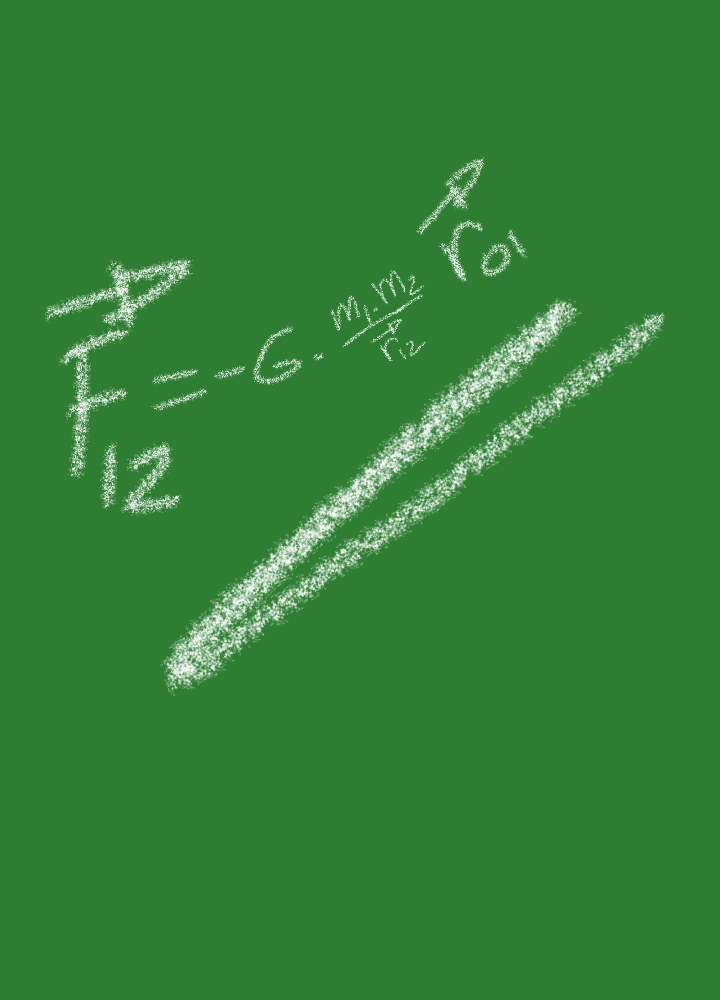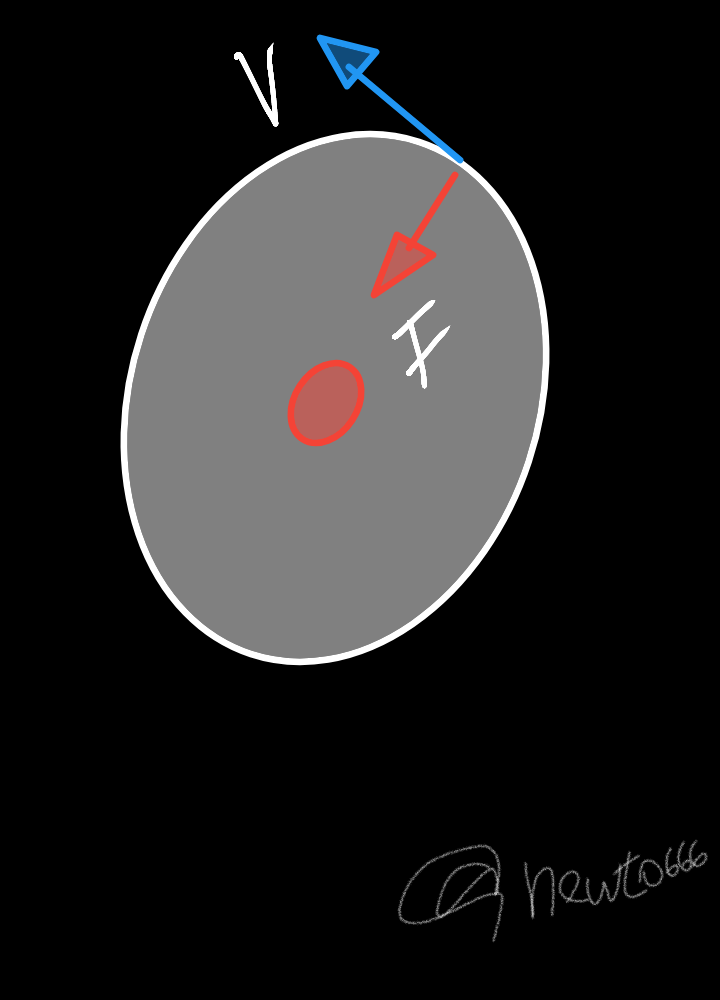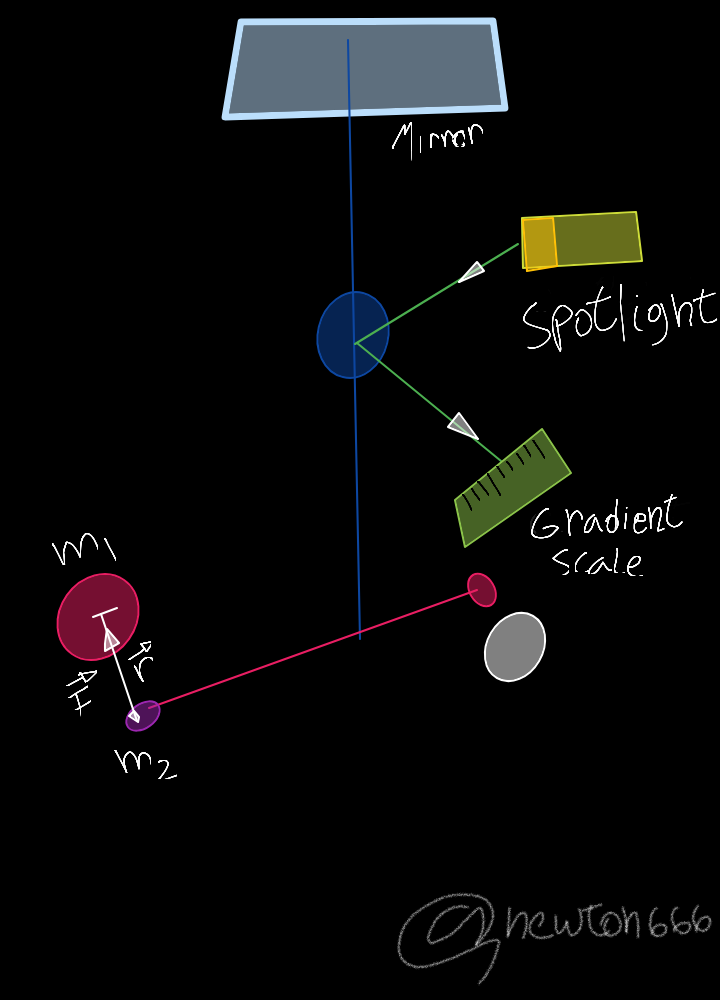# Newton's Universal Law of Gravitation

in StemSocial3 months ago
Greetings colleagues from the Hive community, welcome back to my blog, where you can find content from the wide universe of science, mathematics, biology, physics, chemistry, philosophy, and general knowledge accompanied by scientific outreach.

# Introduction

Starting with common sense what we know about the gravity of our planet Tierrala and this very unique physical phenomenon, what we should know about gravity is simply the attraction between any two masses, where said force is always attractive, and the intensity of the force between two masses inversely depends on the square of their distances, making it an inverse force to the square, in the field of classical philosophy it was considered according to Aristotle they thought that heavy objects fell on the ground faster than the rest, but everything would change thanks to Isaac Newton (1642-1727), the singularity of this context had as its starting point the hypothesis based on Kepler's laws, up to the point of demonstrating the movements of the planets and also of the falling bodies on the surface of the Earth, which were due to the same type of cause. Gravity acts on all matter and that is a function of mass and distance, where every object attracts another object with a force that is proportional to the product of their masses and inversely proportional to the square of the distance that separates them.It is known that the force of attraction between two bodies is inversely proportional to the square of their distance, this formula of the acceleration of gravity is equal to the formula of Newton's law of universal gravitation, if we consider the direct effect of the speed over the gravitational interaction.
Always understood by speed, the speed measured with respect to the natural reference system provided by the kinetic, gravitational or global ether. Information consulted in Law of Global Gravity: Global Physics by José Tiberius, 2016.

Newton's law of universal gravitation mentions: any two bodies attract each other with a force directly proportional to the product of their mass and inversely proportional to the square of the distance that separates them.In this mathematical model, F12 is the force that the mass m1 exerts on m2, ro is the unit vector in the direction and direction of r12 and the minus sign is because F12 and r0 make sense due to the attractive force. . Newton provided us with the foundations of physics and classical kinetics, so he used his hypothesis of the universal law of gravity to describe how it works, unlike the approach proposed by Einstein where he shows us that gravity was a simple curvature in space-time, created by an object with a mass much greater than that of the surrounding objects, but the reality reader friend Newton correctly establishes that the gravity affected all objects with mass in the universe, because it supported quite a lot in the physical meaning of Kepler's three laws on planetary motion.In the following representation it shows us as a rigid rod that has at its ends two small mass one of gold, and that is suspended by its central part by means of a fine quartz thread, in such a way that it approaches two other masses , the gravitational force originates a pair of forces that can be measured by a light beam, a mirror and also a graduated scale, in such a way the force can be calculated by means of a mass balance, which allows determining the constant G with a G value = 6,667.10 ^ -11 Nm ^ 2 / kg ^ 2.Sort:

@tipu curate

Upvoted 👌 (Mana: 24/32)